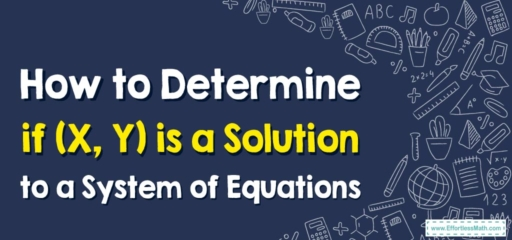# How to Determine if (X, Y) is a Solution to a System of Equations

A system of equations is a set of multiple equations with the same variables. To determine whether a given ordered pair $$(X, Y)$$ is a solution to a system of equations, you need to substitute the values of $$X$$ and $$Y$$ into each of the equations and check if the equations are satisfied. If they are, then the ordered pair is a solution; if they are not, then it is not a solution.## A Step-by-step determining whether (X, Y) is a Solution to a System of Equations

Here is a step-by-step guide on how to do this:

### Step 1: Identify the system of equations

First, you need to have a system of equations. It might look something like this:

Equation 1: $$2x+3y=7$$
Equation 2: $$4x-y=5$$

### Step 2: Identify the ordered pair (X, Y)

Next, you will be given an ordered pair, or you may be asked to determine if a particular pair is a solution. The ordered pair will look something like this: $$(X, Y) = (1, 2)$$.

### Step 3: Substitute the ordered pair into the equations

Now you need to substitute the $$X$$ and $$Y$$ values from the ordered pair into the equations from step 1. The $$X$$ value is substituted for $$x$$, and the $$Y$$ value is substituted for $$y$$.

For our example, this would look like this:

For Equation 1: $$2(1)+3(2)=7⇒ 2+6=7$$, which is not true.
For Equation 2: $$4(1)-2=5⇒ 4-2=5$$, which is also not true.

### Step 4: Check if the equations are true

As you can see, neither equation is true when you substitute the given values of $$X$$ and $$Y$$ into them. This means that the ordered pair $$(1, 2)$$ is not a solution to this system of equations.

If both equations were true after the substitution, then the ordered pair would be a solution to the system of equations.

In summary, to find out if an ordered pair is a solution to a system of equations, you substitute the $$X$$ and $$Y$$ values into each equation in the system. If all equations are satisfied, then the ordered pair is a solution; if not, it is not a solution.

### What people say about "How to Determine if (X, Y) is a Solution to a System of Equations - Effortless Math: We Help Students Learn to LOVE Mathematics"?

No one replied yet.

X
30% OFF

Limited time only!

Save Over 30%

SAVE $5 It was$16.99 now it is \$11.99